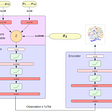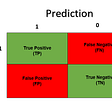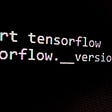# Application of AI in Intrusion Detection System 👨‍💻

Today widely used intrusion detection systems (IDS) are based on different machine learning algorithms. Any IDS system is evaluated by its ability to make the predictions of attacks accurately. Many IDS systems use binary classification which can predict four possible outcomes. Attacks correctly predicted as attacks(TP), or incorrectly predicted as normal(FN). Normal correctly predicted as normal (TN), or incorrectly predicted as an attack (FP).

Before discussing how binary classification is helpful in intrusion detection systems (IDS) let us first understand IDS and Confusion matrix.

# Intrusion Detection System (IDS)

An intrusion detection system (IDS) is a device or software application that monitors a network or systems for malicious activity or policy violations. Any intrusion activity or violation is typically reported either to an administrator or collected centrally using a security information and event management (SIEM) system. A SIEM system combines outputs from multiple sources and uses alarm filtering techniques to distinguish malicious activity.

IDS types can be classified based on single computers to large networks. The most common classifications are network intrusion detection systems (NIDS) and host-based intrusion detection systems (HIDS). A system that monitors important operating system files is an example of a HIDS, while a system that analyzes incoming network traffic is an example of a NIDS.

IDS can also be classified by the detection approach. The most well-known types are signature-based detection (recognizing bad patterns, such as malware) and anomaly-based detection (detecting deviation from a model of “good” traffic, based on machine learning). Another common type is reputation-based detection (recognizing the potential threat according to the reputation scores).

# Confusion Matrix

A confusion matrix is a table that is used to describe the performance (accuracy) of a classification model on a testing dataset for which the true values are known.

## Confusion matrix of binary classification:

Suppose we have the medical data where we have some clinical measurements and we want to apply machine learning methods to them to predict whether or not someone will develop heart disease.

Suppose we used some machine learning model and trained them with training data and now we want to decide what is the accuracy of the model with the help of testing sets.

To find the accuracy of the model on the testing data, we can create the confusion matrix.

The rows in the confusion matrix correspond to what the machine learning algorithm predicted and the columns in a confusion matrix correspond to the known truth (Actual value).

Since there are only two categories to choose from i.e has heart disease & no heart disease. So, the confusion matrix contains only four possibilities and they are called as:

• True Positive: These are the patients that had heart disease that was correctly identified by the model.
• True Negative: These are the patients that did not have heart disease that was correctly identified by the model.
• False Negative: False-negative is when a patient has heart disease, but the model said they didn’t.
• False Positive: False positive are the patients that do not have heart disease, but the model says they do have.

Now we can calculate the accuracy of the model with help of the given formula.

📍Note: Accuracy can be misleading if used with imbalanced datasets, and therefore there are other metrics based on confusion matrix which can be useful for evaluating performance.

# How ML is helpful to improve the performance of IDS?

Nowadays there is an exponential increase in the number of cyber-attacks so the need for an improved Intrusion Detection System(IDS) increased. Despite enormous efforts by the researchers, IDS still faces challenges in improving detection accuracy while reducing false alarm rates and in detecting novel intrusion Machine learning (ML) and deep learning (DL)-based IDS systems are being deployed as potential solutions to detect intrusions across the network in an efficient manner. There are many algorithms available, but the selection of the right algorithm is a very challenging task.

## Artificial Intelligence based IDS

AI-based NIDS most commonly use ML and DL algorithms. Noth ML and DL are broadly classified as supervised and unsupervised algorithms In supervised algorithms, useful information is extracted from the labeled data. While unsupervised algorithms rely on unlabeled data to extract useful features and information

Working of AI based NIDS:

A NIDS based on ML and DL algorithms usually involves the following three major steps: (i) Data preprocessing phase, (ii) Training phase, and (iii) Testing phase. First data is pre-processed to transform it into the format suitable to be used by the algorithms. Then the pre-processed data is divided randomly into two parts, the training dataset, and the testing dataset. The ML or DL algorithms are then trained using the training dataset in the training phase. Once the model is trained, it is tested using the testing dataset and evaluated based on the predictions it made. In the case of NIDS models, the network traffic will be predicted to belong to either normal or attacked class.

## ML Algorithms

ML is a subset of AI that has methods and algorithms which enable the machines to learn automatically using mathematical models in order to extract useful information from large datasets. The most common ML (also called Shallow Learning) algorithms used for IDS are Decision Tree, K-Nearest Neighbor (KNN), Artificial Neural Network (ANN), Support Vector Machine (SVM), K-Mean Clustering.

## Deep learning algorithms

DL is the subset of the ML which includes many hidden layers to get the characteristics of the deep network and it is more efficient than the ML due to its deep structure and ability to learn the important features from the dataset on its own and generate an output.

# Confusion Matrix

These are the most commonly used evaluation metrics for measuring the performance of ML and DL methods for IDS. Confusion Matrix is a two-dimensional matrix providing information about the Actual and Predicted class and includes:

1. True Positive (TP): The data instances correctly predicted as an Attack by the classifier.
2. False Negative (FN): The data instances wrongly predicted as Normal instances.
3. False Positive (FP): The data instances wrongly classified as an Attack.
4. True Negative (TN): The instances correctly classified as Normal instances.

The diagonal of the confusion matrix denotes the correct predictions while nondiagonal elements are the wrong predictions of a certain classifier.

• Precision: It is the ratio of correctly predicted Attacks to all the samples predicted as Attacks.
• Recall: It is a ratio of all samples correctly classified as Attacks to all the samples that are actually Attacks. It is also called a Detection Rate.
• False alarm rate: It is also called the false positive rate and is defined as the ratio of wrongly predicted Attack samples to all the samples that are Normal.
• True negative rate: It is defined as the ratio of the number of correctly classified Normal samples to all the samples that are Normal.
• Accuracy: It is the ratio of correctly classified instances to the total number of instances. It is also called Detection Accuracy and is a useful performance measure only when a dataset is balanced.
• F-Measure: It is defined as the harmonic mean of the Precision and Recall. In other words, it is a statistical technique for examining the accuracy of a system by considering both the precision and recall of the system.

# Conclusion:

With the discussion we can understand how intrusion detection system works which are based on the ML and DL algorithms. Also we can say that Machine learning based intrusion system are good for predicting the attacks but there are many cases where wrong prediction are made e.g: When actual attack is predicted as normal. We can also say that Deep learning approach improves the performance and efficiency of IDS in terms of detection accuracy. Also DL approach have much superior perfomance that the ML-based methods in terms of their ability to learn features by itself and stronger model fitting abilities.

Hope you enjoy reading this blog for more interesting technical stuff connect me on LinkedIn 👇 👇

# Thank You for reading!! 😇😇

--

--

--

I'm passionate learner diving into the concepts of computing 💻

Love podcasts or audiobooks? Learn on the go with our new app.

## Variational Deep Embedding## Mapping Israel's Machine Learning Startups## Cyber Crime with Confusion matrix.## Artificial Intelligence & Everyday Life!## Semantic search at billions scale## The DAP Journey: Multi Programming Language Classifier## TensorFlow 3D -A Quick Introduction## Converting UGATIT to CoreML Model.I'm passionate learner diving into the concepts of computing 💻

## #SpotifyWrapped — using AI and ML is fun## Using TensorFlow 1.x on Ampere architecture GPUs (i.e. RTX 30 series)## Getting Started with ML5.js — Tutorial Part IV: Yoga Pose Detection